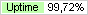Your country: United StatesRUEN

# Equation Solver## News

20.10.13 Version Equation Solver 1.1 released.

## FeedbacksLeave your feedback!
Midlet "Equation Solver" is intended to solve systems of linear algebraic equations with real coefficients of the second and third order (with two and three unknown)
a1*x+b1*y=c1
a2*x+b2*y=c2

a1*x+b1*y+c1*z=d1
a2*x+b2*y+c2*z=d2
a3*x+b3*y+c3*z=d3.

## System Requirements

Mobile phone should have a color screen and maintain a profile MIDP 2.0/CLDC 1.0.
Supported mobile phones

You are using midlet "as is", at your own risk.Midlet is distributed in the form of ZIP-archive containing the files EqSolver.jad and EqSolver.jar,  designed for installation on the phone with the appropriate software  (Nokia PC Suite for Nokia,  Siemens Data Exchange Software for Siemens, Sony Ericsson PC Suite for Sony Ericsson etc.).

Checksum ZIP-archive MD5: 0c1b7d81fc03fe792cc05c79e525859e - 1.1

## Features

When you run the midlet splashscreen appears(within 3 seconds), showing where you can stop to press any key. After the splashscreen, open the main form of midlet:By default midlet is set to decide the third-order systems of equations and equation coefficients are assigned zero value. To install a different dimension or reset coefficients need to select the appropriate dimension of the matrix of coefficients, and then click.
Writing the coefficients is performed in the following order: a1, b1, c1, (d1), a2, b2, c2, (d2), a3, b3, c3, (d3). To enter, you must enter the value in the box, which shows the input symbol coefficient, and click. This input field is automatically configured to input following coefficient (in the box displays the previous value, which can be changed or unchanged). Writing the coefficients of a cyclically repeated (after entering the coefficient values c3 (d3) midlet is set to enter the coefficient values a1 ).
To enter a minus sign is necessary to remove the numbers in cell entry and press the "*" for a decimal point must be to press "#" (in different models of phones required keys may vary).
Once the coefficients of the system to solve the system, click.If the system has a solution, the 1 second window appears with the message of the successful solution of the systemand the basic form midlet solution of the system is shown (values of unknown variables). If the system has no solutions, the 1 second window appears with the error message, and in the main form instead of the unknown variables are displayed question marks.
To solve the following system is required to change the dimension of the system (if necessary) and add new or adjust the previous value of the coefficients.
To display information about the midlet, click.
To close midlet, click.

## Test solution

The system of equations of second order
2*x-1*y=-3.25
-3*x+0.5*y=1.5
Solution (MathCad):Solution (Equation Solver):The system of equations of third order
10*x-2*y-8*z=-72
2*x+5*y+10*z=37
3*x+10*y-0.5*z=6.25.
Solution (MathCad):Solution (Equation Solver):## ScreenshotsSun Java WTK Emulator

## Whats new in Equation Solver 1.1

* changed the website address of the midlet

### Contacts### © 2008 - 2014 Alexey "FoxyLab" Voronin